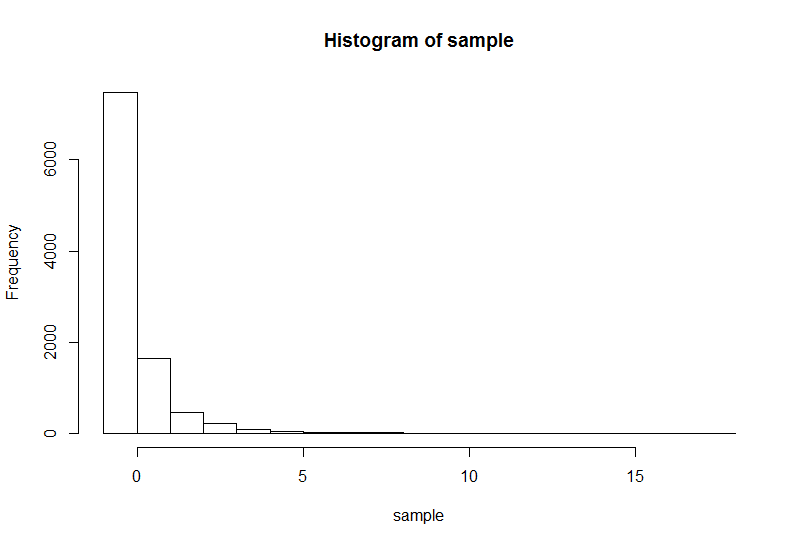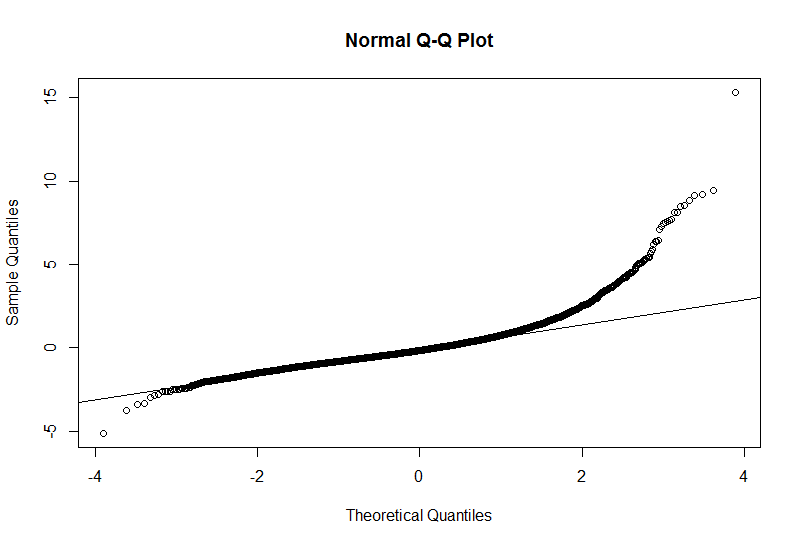Sunday, October 30, 2016

No, a breach of normality probably won't cause a 17% Type 1 error rate

Cain, M. K., Zhang, Z., & Yuan, K.-H. (2016). Univariate and multivariate skewness and kurtosis for measuring nonnormality: Prevalence, influence and estimation. Behavior Research Methods. https://doi.org/10.3758/s13428-016-0814-1

From the abstract:
"this study examined 1,567 univariate distriubtions and 254 multivariate distributions collected from authors of articles published in Psychological Science and the American Education Research Journal. We found that 74 % of univariate distributions and 68 % multivariate distributions deviated from normal distributions. In a simulation study using typical values of skewness and kurtosis that we collected, we found that the resulting type I error rates were 17 % in a t-test and 30 % in a factor analysis.  Hence, we argue that it is time to routinely report skewness and kurtosis..."

Hang on... a 17% Type I error rate purely due to non-normality? This seemed implausibly high to me. In an OLS model such as a t-test (or ANOVA, or ANCOVA, or regression, or whatever), the assumption of normally distributed error terms really isn't very important. For example, it isn't required for the estimates to be unbiased or consistent or efficient. It is required for the sampling distribution of test statistics such as the t statistic to follow their intended distributions, and thus for significance tests and confidence intervals to be trustworthy... but even then, as the sample size grows larger, as per the CLT these sampling distributions will converge toward normality anyway, virtually regardless of the original error distribution. So the idea of non-normal "data" screwing up the Type 1 error rate to this degree was hard to swallow.

So I looked closer, and found a few things that bugged me about this article. Firstly, in an article attempting to give methodological advice, it really bugs me that the authors misstate the assumptions of OLS procedures. They say:

" we conducted simulations on the one-sample t-test, simple regression, one-way ANOVA, and confirmatory factor analysis (CFA).... Note that for all of these models, the interest is in the normality of the dependent variable(s)."

This isn't really true. We do not assume that the marginal distribution of the dependent variable is normal in t-tests, regression, or ANOVA. Rather we assume that the errors are normal. This misconception is something I've grumbled about before. But we can set this aside for the moment given that the simulation from the article that I'll focus on pertains to the one-sample t-test, where the error distribution and the marginal distribution of the data coincide.

So let's move on to looking at the simulations of the effects of non-normality on Type I error rates for the one-sample t-test (Table 4 in the article). This is where the headline figure of a "17%" Type 1 error rate comes from, albeit that the authors seem to be mis-rounding 17.7%. Basically what the authors did here is:
• Estimate percentiles of skewness and (excess) kurtosis from the data they collected from authors of published psychology studies
• Use the PearsonDS package to repeatedly simulate data from the Pearson family of distributions under different conditions of skewness and sample size
• Run one-sample t-tests on this data.
There are a number of conditions they looked at, but the 17.7% figure comes about when N = 18 and skewness = 6.32 (this is the 99th percentile for skewness in the datasets they observed).

However,  they also note that "Because kurtosis has little influence on the t-test, it was kept at the 99th percentile, 95.75, throughout all conditions." I'm unsure where they get this claim from: to the extent that non-normality might cause problems with Type 1 error, both skewness and kurtosis could do so. On the face of it, this is a strange decision: It means that all the results for the one-sample t-test simulation are based on an extremely high and unusual level of kurtosis, a fact that is not emphasised in the article.

As it happens, one of the real reasons why they chose this level of kurtosis is presumably that the simulation simply just wouldn't run if they tried to combine a more moderate degree of kurtosis with a high level of skewness like 6.32. The minimum possible kurtosis for any probability distribution is skewness^2 + 1.

That technical note aside, the net effect is that the headline figure of a Type I error rate of 17% is based on a tiny sample size (18) and an extremely unusual degree of non-normality: An extremely high degree of skewness (6.32, the 99th percentile for skewness across their observed datasets), and an extremely high degree of excess kurtosis (95.75, again the 99th percentile). To give you an idea of what this distribution looks like, check the histogram below (10,000 data points drawn from this distribution). It's a little hard to imagine even a very poor researcher not noticing that their is something amiss if this was the distribution of their raw data!In fact, it's worth noting that the authors "contacted 339 researchers with publications that appeared in Psychological Science from January 2013 to June 2014 and 164 more researchers with publications that appeared in the American Education Research Journal from January 2010 to June 2014", but we have no idea how many of these authors applied analyses that actually assumed normality. No doubt, some of the extreme levels of skewness and kurtosis arose in cases such as reaction time measurements or count data, where even without checking diagnostics the original authors might have been well aware that a normality assumption was inappropriate.

So what about more plausible degrees of non-normality? If anything, a close read of the article shows how even quite dramatic non-normality causes few problems: For example, take the case of N = 18, skewness of 2.77 and excess kurtosis of 95.75 (a small sample size with still quite extreme non-normality, as visualised in the q-q plot below - albeit not as extreme as the case discussed previously). The authors find that the Type 1 error rate (with alpha of 0.05) in this scenario is... 6.4%. That's hardly something to get too stressed out about!Ok, so non-normality only really causes Type I problems in extremely severe situations: E.g., pathologically high levels of skewness and kurtosis combined with small sample sizes. But why am I picking on the authors? There is still at least some small danger here of inflated Type 1 error - isn't it good to be conservative and check for these problems?

Well, I have two main worries with this perspective.

Firstly, it's my perception that psychologists worry far too much about normality, and ignore the other assumptions of common OLS procedures. E.g., in descending order of importance:
• Conditional mean of errors for any combination of predictors are assumed to be zero (breached if correlated measurement error present, or any measurement at all in the Xs, or randomisation not conducted, or unmodelled non-linear relationships present between Xs and Y)
• Error terms are assumed to be independent
• Error terms are assumed to have the same variance, regardless of the levels of the predictors.

Now breaches of some of these assumptions (especially the first one) are much more serious, because they can result in estimates that aren't unbiased and consistent and efficient; consequently Type I error rates can of course be substantially inflated (or deflated). As methodologists, we need to bang on about normality less, and the other assumptions more. As Andrew Gelman has said, normality is a minor concern in comparison to some of the other assumptions (note that his phrasing of the OLS/regression assumptions is a little different to how I've written them above, though the ideas are similar).

Secondly, I worry that by encouraging researchers to check for non-normality (and then potentially change their analysis method if problems are found), we introduce extra researcher degrees of freedom into the analysis process. Deciding whether error non-normality is present is a subjective and challenging task, and there are many potential solutions to non-normality researchers can try. It thus strikes me that the potential for selective reporting (and even p-hacking) involved in encouraging researchers to check for non-normality and then attempt various remedies is actually a much greater threat to validity than any direct effect of non-normality.

In conclusion...Non-normality can cause problems, but only in very unusual situations. If you're running a t-test or regression, chances are that there are more important things for you to be worrying about.

1.Great article! Would you be able to clarify this section for me:

"No doubt, some of the extreme levels of skewness and kurtosis arose in cases such as reaction time measurements or count data, where even without checking diagnostics the original authors might have been well aware that a normality assumption was inappropriate".

Why would a normality assumption be inappropriate for reaction time measurements?

1.Hi James, thanks for the kind words!

On second thought, given the limited importance of the normality assumption, "inappropriate" is maybe a bit strong. I was reflecting on the fact that there are certain types of data with which researchers would typically *not* use methods that assume normal errors, simply because more well-suited parametric methods are available. Count data is one example - a researcher analysing a count DV might use a Poisson or negative binomial model instead.

Reaction time was an example that jumped to mind for me because the distribution with M=0, SD=1, skewness = 6.32 and excess kurtosis = 95.75 (in the histogram above) looks like it could possibly arise when measuring reaction times. I.e., many reaction times may be very close to zero, with a handful that are much larger. As Jonas mentions, researchers using reaction times as the DV will often assume something other than a normal distribution for the errors (e.g., perhaps a gamma distribution).

Personally I think that the data collection aspect of the Cain et al. article could perhaps have been improved a bit if they'd restricted their search to data where a normality assumption had actually been made by the original researchers.

2.RT measurements are typically right-skewed. They are more correctly described as gamma distributed, I think.